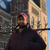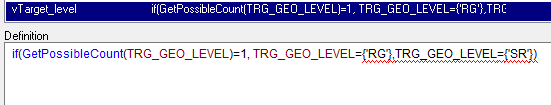# QlikView App Dev

Discussion Board for collaboration related to QlikView App Development.

Announcements
Skip the ticket, Chat with Qlik Support instead for instant assistance.
cancel
Showing results for
Did you mean:Anonymous
Not applicable

## Variable in Set Analysis

Hi experts!

I am trying to define a variable that will be used in several expressions. This variable has a set analysis in it which also has a variable inside. It is like this:

vTarget_level = if(GetPossibleCount(TRG_GEO_LEVEL)=1, TRG_GEO_LEVEL={'RG'},TRG_GEO_LEVEL={'SR'});

vTargetCSAT_TablChart= sum({<TRG_CODE={'CSAT'}, \$(=vTarget_level)>} TRG_TARGET * TRG_Target_Weights)/sum({<TRG_CODE={'CSAT'},\$(=vTarget_level)>}TRG_Target_Weights)

I use it on a text object as:

=\$(vTargetCSAT_TablChart)

What gives error. If i put in the text box:

=(vTargetCSAT_TablChart)

Results: sum({<TRG_CODE={'CSAT'},>} TRG_TARGET * TRG_Target_Weights)/sum({<TRG_CODE={'CSAT'},>}TRG_Target_Weights)

How should I do it so it gives the result?? Thanks.4 RepliesChampion

Hi,

verify that you didn't put an '=' when declaring both variables.

and use this:

=\$(vTargetCSAT_TablChart)Anonymous
Not applicable
Author

There is no '=' in the declaration. They are both like thisChampion

Actually i didn't read the content of the variables and the association between them.

did you try your first variable before ? the output should be a stringMaster II

try this

vTarget_level=  if(GetPossibleCount(TRG_GEO_LEVEL)=1, 'TRG_GEO_LEVEL={'RG'}','TRG_GEO_LEVEL={'SR'}');

not put it in a text box and check

Learning never stops.Community Browser